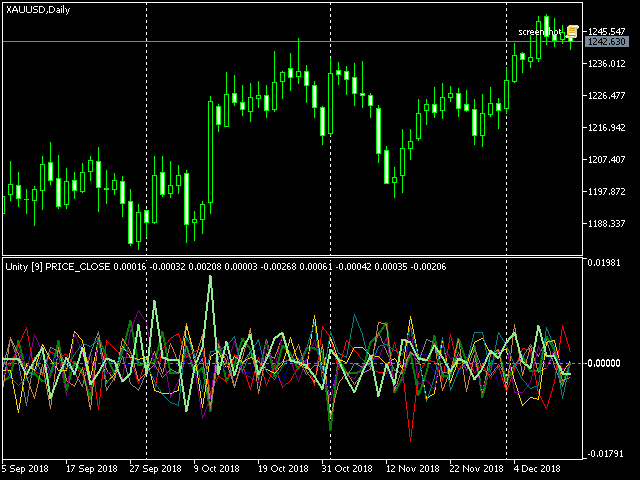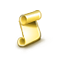Watch how to download trading robots for free

Interesting script?
So post a link to it -
let others appraise it

You liked the script? Try it in the MetaTrader 5 terminal# Unity - indicator for MetaTrader 5

Views:
2200
Rating:
votes: 13
Published:
2019.07.17 16:38

Unity is a cluster multi-currency indicator showing synchronous changes in the value of metals and major currencies in a generalized form. It was first published in the article "Practical Use of Kohonen Neural Networks in Algorithmic Trading. Part II. Optimizing and forecasting".

In the essence its work is described by the following algorithm. Consider it on the minimalistic example with one currency pair (EURUSD) and gold (XAUUSD).

At every moment, the market state (that is current prices, or open prices of the bars) is expressed by obvious formulae:

EUR / USD = EURUSD

XAU / USD = XAUUSD

where variables EUR, USD, XAU are pure "values" of the assets, and EURUSD, XAUUSD are constants (exchange rates, or quotes).

To find the variables, we supplement the system with another equation, limiting the sum of the squares of the variables to unity:

EUR*EUR + USD*USD + XAU*XAU = 1

Hence the name of the indicator — Unity.

Simple substitution gives:

EURUSD*USD*EURUSD*USD + USD*USD + XAUUSD*USD*XAUUSD*USD = 1

Then USD can be found as:

USD = sqrt(1 / (1 + EURUSD*EURUSD + XAUUSD*XAUUSD))

and all other variables can be calculated.

In general:

x0 = sqrt(1 / (1 + sum(C(xi, x0)**2))), i = 1..n

xi = C(xi, x0) * x0, i = 1..n

where n — number of variables, C(xi,x0) — the quote of i-th pair including corresponding variables. Please note, that the number of variables is 1 more than instruments.

Since the coefficients C involved in the calculation are quotes that are usually very different, in the indicator they are additionally multiplied by contract's sizes: this gives more or less comparable values (at least, of one order). To see them in the indicator window (just for information) there is an AbsoluteValues input parameter that should be set to true. By default, it is, false, of course, and the indicator calculates the increments of variables:

yi = xi0 / xi1 - 1,

where xi0 and xi1 are values on the last and the previous bars respectively.

Here is the screenshot for the cluster of EURUSD,GBPUSD,USDCHF,USDJPY,AUDUSD,USDCAD,NZDUSD,XAUUSD:Cluster multi-currency indicator Unity, XAUUSD

The lines of assets that make up work symbol of the current chart (in this case, XAU and USD) are shown thick, the rest are thin.

The main input parameters of the indicator:

• Instruments — the string with the names of work symbols separated by commas; it is necessary for all instruments to have one common currency - either base or quoting one;
• BarLimit — number of bars to calculate;
• Draw - drawing style;
• PriceType - price type used in calculations;
• SaveToFile — (option) a name of csv-file to which the indicator exports values for analysis in an external program, for example, in a neural network; the structure of the file is the following: the first column is the date, all subsequent ones are the values of the corresponding indicator buffers;
• ShiftLastBuffer - (option) flag for switching the mode in which the csv-file is formed; when the option is false, the data of the same bar are stored in the file on each line, the number of columns is equal to the number of instruments plus one due to the division of tickers into components, and plus one more — the first one — with dates, column names correspond to currencies and metals; when the option is true, an additional column called FORECAST is created, in which the values from the column with the last asset are saved with the shift to one bar forward; thus, in each row we see not only all the data for the current bar, but also next value of the last variable, which can be used by forecasting algorithms.

Recommended timeframes - D1 and higher.PriceSpeed

This indicator shows average and peak speeds of price changes per minute.RSI candles - lite ressource

Improved version based on RSI_candles by © mladen 2018 https://www.mql5.com/en/code/20968Export Calculated Prices & Indicator Buffers

Export tool for OHLC prices, calculated prices & indicator buffersMAxCD

Three Moving Averages Convergence/Divergence - Indicator for MT5